Courses

# Angle Modulation & Demodulation Notes | EduRev

## GATE : Angle Modulation & Demodulation Notes | EduRev

The document Angle Modulation & Demodulation Notes | EduRev is a part of the GATE Course Communication System.
All you need of GATE at this link: GATE

Angle Modulation:

• Angle modulation may be defined as the process in which the total phase angle of a carrier wave is varied in accordance with the instantaneous value of the modulating or message signal while keeping the amplitude of the carrier constant.
• There are two types of angle modulation schemes as under
• Phase Modulation (PM)
• Frequency Modulation (FM)
• These modulation schemes are also called as non-linear modulation schemes.

(i) Phase Modulation (PM):

• PM is that type of angle modulation in which the phase angle φ is varied linearly with a baseband or modulating signal x(t) about an unmodulated phase angle: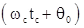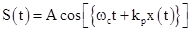where kp = Phase sensitivity of the modulator

(ii) Frequency Modulation (FM):

FM is that type of angle modulation in which the instantaneous frequency ωi is varied linearly with a message or baseband signal x(t) about an unmodulated frequency ωc.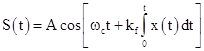where, kf = Frequency sensitivity of the modulator

(iii) Representation of FM and PM Signals:

An angle modulated signal in general can be written as

S(t) = Ac cos [θ(t)]

where, θ (t) is the phase of the signal and its instantaneous frequency is given by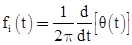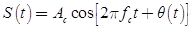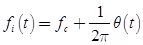If m(t) is the message signal, then in PM system, we have

For FM system, we have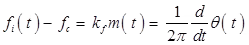where, kp and kf are phase and frequency deviation constants respectively.Key Points:

• By passing message through a differentiator, then through a FM modulator we get PM modulated signal.
• By passing message through a integrator and then PM modulator we get FM modulated signal.

(iv) PM and FM Modulated Signals for Sinusoidal Message Signal: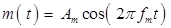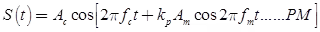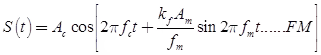The maximum change in instantaneous frequency from the average frequency ωc is called frequency deviation which depends upon the magnitude and sign of km(t). Frequency deviation: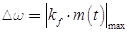Carrier Swing: The total variation in frequency from the lowest to the highest point is called carrier swing.

The carrier swing = 2 x frequency deviation  = 2 x Δω

Key Points:

• The amount of frequency deviation or variation depends upon the amplitude (loudness) of the modulating (audio) signal. This means that louder the sound, greater the frequency deviation and vice-versa.
• The frequency deviation is useful in determining the FM signal bandwidth.
• In FM broadcast, the highest audio frequency transmitted is 15 kHz.

Modulation Index: For FM, the modulation index is defined as the ratio of frequency deviation to the modulating frequency.

mf = frequency deviation / modulating frequency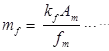for FM (sinusoidal message signal)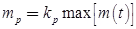for PM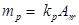for PM (sinusoidal message signal)

Note: The mf may be greater than unity.

Per cent Modulation: The term 'per cent modulation as it is used in reference to FM' refers to the ratio of actual frequency deviation to the maximum allowable frequency deviation.

Per cent modulation :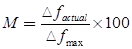where,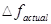is  Actual frequency deviation,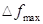is Maximum frequency deviation

In per cent modulation, the following features will be considered

• Maximum modulating frequency is 15 kHz.
• Maximum frequency deviation is ± 75 kHz.
• Frequency stability of the carrier is ± 2 kHz.
• Allowable bandwidth per channel is 200 kHz.

(iv) Types of FM:

The FM signal can be classified based on sensitivity constant as:

• Narrow Band FM: In this case, kf is small and hence the bandwidth of FM is narrow.
• Wide Band FM: In this case, kf is large and hence the FM signal has a wide bandwidth.

(v) Another Representation of PM and FM Signals for Sinusoidal Message: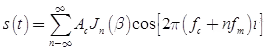where β is modulation index. In (β) is known as Bessel function of first kind of order n. Total bandwidth requirement for angle modulated signal is infinite. Basically the effective bandwidth is the separation between the two extreme significant side frequencies on either side of the carrier.

Bandwidth of the Angle Modulated Signal: In general, the effective bandwidth of an angle modulated signal, which contains atleast 98% of the signal power is given by.

Bc = 2 (β + 1) fm is also known as Carson's formula

where, β is modulation index.

For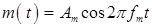Number of harmonics in the bandwidth (including the carrier) is: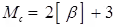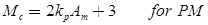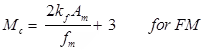• By seeing the graph of angle modulation signal we can't tell whether it is PM or FM.
• FM receiver may be fitted with amplitude limiters to remove the amplitude variations caused by noise.

Relation between PM and FM:

PM signal: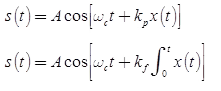(vi) Generation of FM Using Phase Modulator: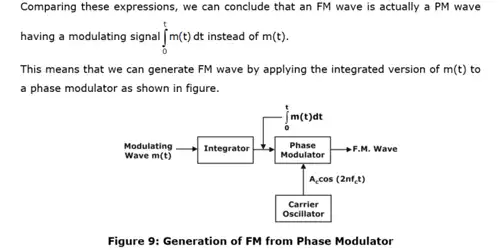(vii) Generation of PM using a Frequency Modulator:

It is also possible to generate a PM wave using a frequency modulator as shown in figure 3. The modulating signal is first passed through a differentiator and then applied to a frequency modulator.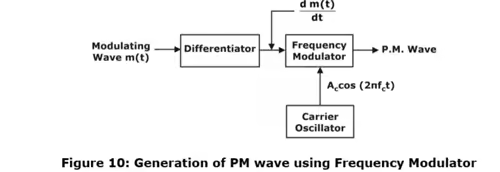This discussion has revealed that it is possible to obtain all the properties of FM from a PM wave and vice versa. At the output of the differentiator, we get the differentiated version of modulating signal i.e. dm(t)/dt. Hence, output of the frequency modulator will be,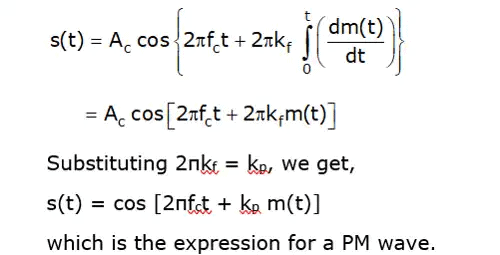(viii) FM Over AM:

• It is possible to reduce noise still further by increasing the frequency deviation but in AM this is not possible.
• Standard frequency allocations provide a guard band between commercial FM stations. Due to this, there is less adjacent channel interference than in AM.
• FM broadcasts operate in the upper VHF and UHF frequency ranges at which there happens to be less noise than in the MF and HF ranges occupied by AM broadcasts.

The amplitude of the FM wave is constant. It is thus independent of the modulation depth, whereas in AM, modulation depth governs the transmitted power.

Note: A much wider channel typically 200 kHz is required in FM as against only 10 kHz in AM broadcast. This form serious limitation of FM.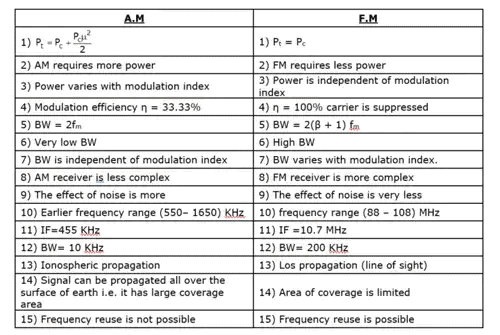(ix) Generation of FM:

The FM modulator circuits used for generating FM signals may be put into two categories as under

• The direct method or parameter variation method.
• The indirect method or the Armstrong method.

Direct Method

This method is most widely used for generation of WBFM signal.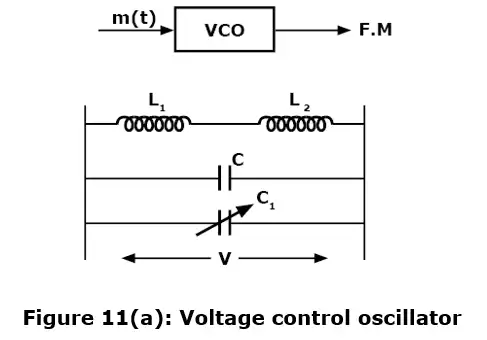Drawbacks of Direct Method for FM Generation

Following are the drawbacks of the direct method:

1. In direct method of FM generation, it is not easy to get a high order stability in carrier frequency. This is due to the fact that generation of carrier signal is directly affected by the baseband or modulating signal. The baseband signal directly controls the tank circuit of the carrier generator and thus a stable oscillator circuit (i.e. crystal oscillator) cannot be used. This means that the carrier generation cannot be of high stability which is a necessary requirement. A solution to this problem is the indirect method i.e. Armstrong method of FM generation. In fact, in this method, the carrier oscillator does not respond to the modulating signal directly rather the generation of the carrier is made isolated from other parts of the circuit. Thus, stable crystal oscillators may be used to generate carrier signal.
2. The non-linearity of the varactor diode produces a frequency variation due to harmonics of the modulating or baseband signal and therefore the FM signal is distorted. We will have to take the proper cares to keep this type of distortion minimum.

Despite all above drawbacks, the direct method is utilized for high power FM generation in several applications.

[Note: A low-pass filter compensates for higher phase shift and frequency deviation at the higher modulating frequencies to produce indirect FM.]

(x) ARMSTRONG METHOD: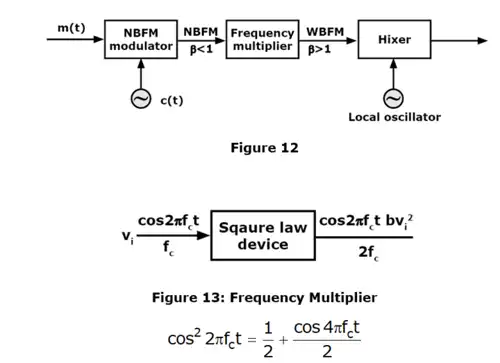DC component is eliminated using an amplifier of gain 2 then o/p = cos 4πfct. when one more square law device is connected frequency gets multiplied again by 2. Thus 2 square law devices in cascade multiplies the frequency by 4.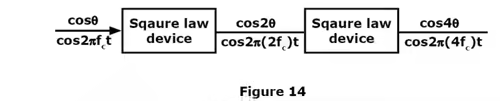Assume that the message signal and carrier are applied to a NBFM modulate. The output signal is Ac cos [2πfct + βsin 2πfmt]. If the signal is passed through frequency multiplier the final output is Ac cos [n(2π fct + βsin 2πfmt)]. In a frequency multiplier, carrier frequency and β are increased by a factor of n. But the message frequency is same. (As multiplier changes the carrier frequency, it must be brought back to the original carrier frequency. This is done by using a mixer)

(xi) Demodulation of FM:

The demodulation process of FM waves is exactly opposite to that of the frequency modulation. The AM detector is nothing but a rectifier, but FM demodulator is a frequency to amplitude converter. It is necessary to convert the frequency variations in FM wave at its input into amplitude variations at its output to recover the original modulating signal.

(xii) Requirements of FM demodulator (Detector):

The FM demodulator must satisfy the following requirements:

1. It must convert frequency variations into amplitude variations.
2. This conversion must be linear and efficient.
3. The demodulator circuit must be insensitive to amplitude changes. It should respond only to the frequency changes.
4. Its adjustment and operation should not be critical.

Schematic of an FM demodulator based of FM to AM conversion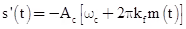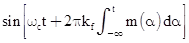(xiii) Performance comparison of FM & PM System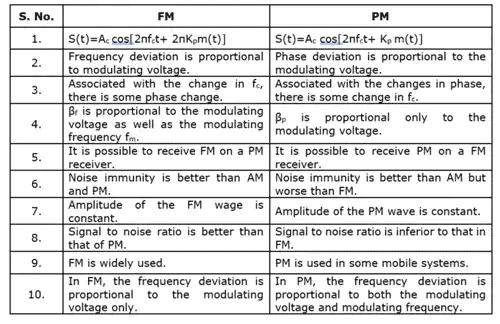Offer running on EduRev: Apply code STAYHOME200 to get INR 200 off on our premium plan EduRev Infinity!

10 docs|12 tests

,

,

,

,

,

,

,

,

,

,

,

,

,

,

,

,

,

,

,

,

,

;